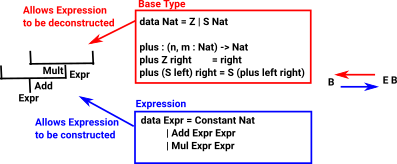# Maths - Discrete Algebraic Structures

We have already looked at groups and on these pages we look at other algebraic structures.

For a more general discussion of algebras see the page here.

### F-AlgebrasAn algebra consists of: A data type - an underlying type An expression - a tree structure where the leaves are elements of this data type. A way to evaluate expressions

If the datatype is denoted 'A' then going one layer up in this tree structure can be denoted: F(A).

So to evaluate an expression we need a function α: F(A) -> A

### Computing with AlgebrasIf we have a 'base type' such as Nat we can construct an algebra from that. So 'Expr' builds up a tree structure and the base type allows us to contract it down again.

 We can expand Expr to include variables like this: In this case we can't always reduce the expression to a single value. In many cases we would use de Bruijn notation rather than naming the variable with a string. ```data Expr = Constant Nat | Var String | Add Expr Expr | Mul Expr Expr```

### Fixpoints of Expression

An expression is defined recursively so an expression can exist inside an expression and so on. Otherwise we could have say 1+6 but not 1 + (2 * 3).

So an expression of an expression is still an expression, that is:

Expr X = Expr (Expr X)

This is called a fixed point often contracted to fixpoint.

### Existence of Fixpoints - Monotonicity

A fixpoint is guaranteed to exist if the function is monotone (Knaster-Tarski fixpoint theorem).

That is, if there is an order on the elements and the function preserves the order.

This applies even if the order is a partial order provided there is a bottom element.

This order may be thought of in different ways depending if we are coming from the direction of denotional semantics or category theory:

Denotional Semantics Category Theory
Order Lower means less well defined and higher means more defined. Lower means toward 'initial' and higher means toward 'final'.
Bottom Element Denoted. This means undefined/error/non-terminating computation. The least fixed point is the fixed point with a morphism to every other morphism.

The semantics should not just be monotone but also continuous , in which the least fixpoint is the union of all finite iterates.

Functions for which f() =are called strict.

### Strictly Positive Functor

An inductive type is defined by a functor but not all functors can be used to define an inductive type.

A functor is strictly positive if it is defined by an expression that only uses constant types, the variable type X and the operators ×, +, ->, so that X only occurs to the right of arrows. Arrows (functions) are covarient in codomain and contravarient in domain.

### Non-Associative Algebras

Where I can, I have put links to Amazon for books that are relevant to the subject, click on the appropriate country flag to get more details of the book or to buy it from them.The Princeton Companion to Mathematics - This is a big book that attempts to give a wide overview of the whole of mathematics, inevitably there are many things missing, but it gives a good insight into the history, concepts, branches, theorems and wider perspective of mathematics. It is well written and, if you are interested in maths, this is the type of book where you can open a page at random and find something interesting to read. To some extent it can be used as a reference book, although it doesn't have tables of formula for trig functions and so on, but where it is most useful is when you want to read about various topics to find out which topics are interesting and relevant to you.